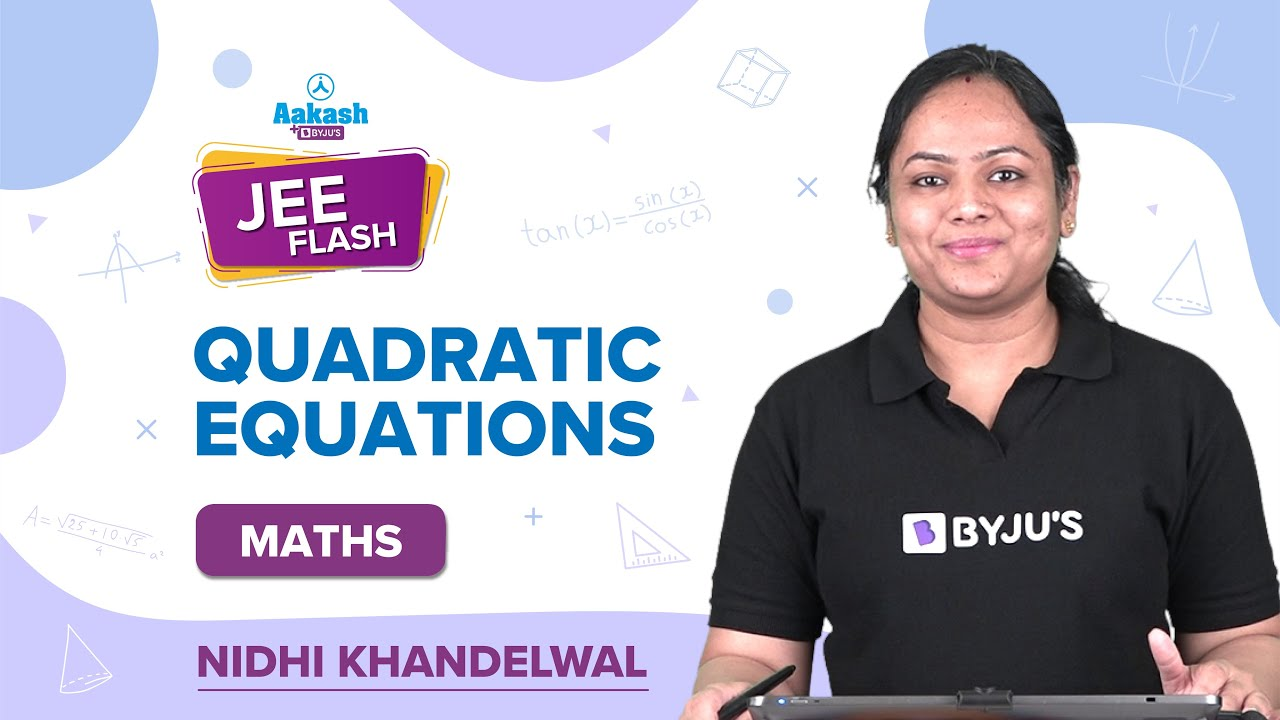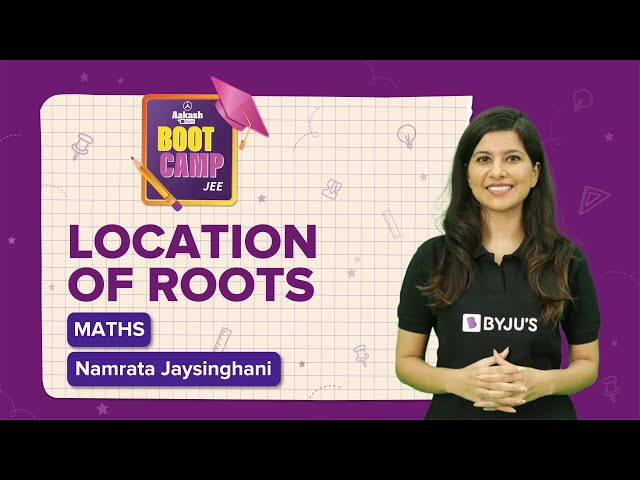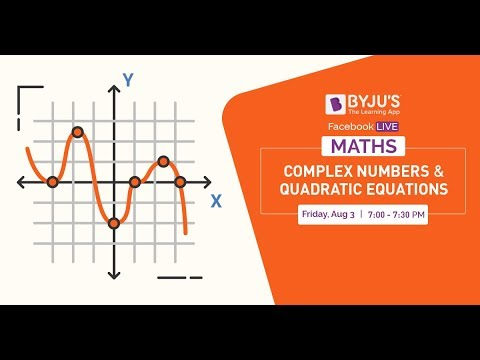Win up to 100% scholarship on Aakash BYJU'S JEE/NEET courses with ABNAT Win up to 100% scholarship on Aakash BYJU'S JEE/NEET courses with ABNAT

# Complex Numbers and Quadratic Equations Previous Year Questions With Solutions

Get Complex Numbers and Quadratic Equations previous year questions with solutions here. All solutions are prepared by subject matter experts of Mathematics at BYJU’S. The questions in the article enable the students to predict the difficulty level of the questions in the upcoming JEE Main and JEE Advanced exams. The chapter contains important concepts such as, algebra of complex numbers, modulus and argument, complex conjugate, properties of complex numbers, square root of complex numbers and complex equations, De-moivre’s theorem, Vector representation and rotation of complex numbers and many more.

## JEE Main Maths Complex Numbers & Quadratic Equations Previous Year Questions With Solutions

Question 1: If (1 + i) (1 + 2i) (1 + 3i) ….. (1 + ni) = a + ib, then what is 2 * 5 * 10….(1 + n2) is equal to?

Solution:

We have (1 + i) (1 + 2i) (1 + 3i) ….. (1 + ni) = a + ib …..(i)

(1 − i) (1 − 2i) (1 − 3i) ….. (1 − ni) = a − ib …..(ii)

Multiplying (i) and (ii),

we get 2 * 5 * 10 ….. (1 + n2) = a2 + b2

Question 2: If z is a complex number, then the minimum value of |z| + |z − 1| is ______.

Solution:

First, note that |−z|=|z| and |z1 + z2| ≤ |z1| + |z2|

Now |z| + |z − 1| = |z| + |1 − z| ≥ |z + (1 − z)|

= |1|

= 1

Hence, minimum value of |z| + |z − 1| is 1.

Question 3: For any two complex numbers z1 and z2 and any real numbers a and b; |(az1 − bz2)|2 + |(bz1 + az2)|2 = ___________.

Solution:

|(az1 − bz2)|2 + |(bz1 + az2)|2

$$\begin{array}{l}= (az_{1}-bz_{2})(a\overline{z_{1}}-b\overline{z_{2}})+(bz_{1}+az_{2})(b\overline{z_{1}}+a\overline{z_{2}})\end{array}$$

= (a2 + b2) (|z1|2 + |z2|2)

Question 4: Find the complex number z satisfying the equations

$$\begin{array}{l}|\frac{z-12}{z-8i}| = \frac{5}{3},\ |\frac{z-4}{z-8}| = 1.\end{array}$$

Solution:

We have

$$\begin{array}{l}|\frac{z-12}{z-8i}| = \frac{5}{3}\end{array}$$
,
$$\begin{array}{l}|\frac{z-4}{z-8}| = 1\end{array}$$

Let z = x + iy, then

$$\begin{array}{l}|\frac{z-12}{z-8i}| = \frac{5}{3}\end{array}$$

⇒ 3|z − 12| = 5 |z − 8i|

3 |(x − 12) + iy| = 5 |x + (y − 8) i|

9 (x − 12)2 + 9y2 = 25x2 + 25 (y − 8)2 ….(i) and

$$\begin{array}{l}|\frac{z-4}{z-8}| = 1\end{array}$$

⇒ |z − 4| = |z − 8|

|x − 4 + iy| = |x − 8 + iy|

(x − 4)2 + y2 = (x − 8)2 + y2

⇒ x = 6

Putting x = 6 in (i), we get y2 − 25y + 136 = 0

y = 17, 8

Hence, z = 6 + 17i or z = 6 + 8i

Question 5: If z1 = 10 + 6i, z2 = 4 + 6i and z is a complex number such that

$$\begin{array}{l}amp\ \frac{z-z_1}{z−z_2} = \frac{\pi}{4}\end{array}$$
, then the value of |z − 7 − 9i| is equal to _________.

Solution:

Given numbers are z1 = 10 + 6i, z2 = 4 + 6i and z = x + iy

$$\begin{array}{l}amp\ \frac{z-z_1}{z−z_2} = \frac{\pi}{4}\end{array}$$

amp [(x − 10) + i (y − 6) (x − 4) + i (y − 6)] = π / 4

$$\begin{array}{l}\frac{(x − 4) (y − 6) − (y − 6) (x − 10)}{(x − 4) (x − 10) + (y − 6)^2}= 1\end{array}$$

12y − y2 − 72 + 6y = x2 − 14x + 40 …..(i)

Now |z − 7 −9i| = |(x − 7) + i (y − 9)|

From (i), (x2 − 14x + 49) + (y2 − 18y + 81) = 18

(x − 7)2 + (y − 9)2 = 18 or

[(x − 7)2 + (y − 9)2]½ = ½ = 3√2

|(x − 7) + i (y − 9)| = 3√2 or

|z − 7 −9i| = 3√2.

Question 6: Suppose z1, z2, z3 are the vertices of an equilateral triangle inscribed in the circle |z| = 2. If z1 = 1 + i√3, then find the values of z3 and z2.

Solution:

One of the numbers must be a conjugate of z1 = 1 + i√3 i.e. z2 = 1 − i√3 or z3 = z1 ei2π/3 and

z2 = z1 e−i2π/3 , z3 = (1 + i√3) [cos (2π / 3) + i sin (2π / 3)] = −2

Question 7: If cosα + cos β + cos γ = sin α + sin β + sin γ = 0 then what is the value of cos 3α + cos 3β + cos 3γ?

Solution:

cos α + cos β + cos γ = 0 and sin α + sin β + sin γ = 0

Let a = cos α + i sin α; b = cos β + i sin β and c = cos γ + i sin γ.

Therefore, a + b + c = (cosα + cosβ + cosγ) + i (sinα + sinβ + sinγ) = 0 + i0 = 0

If a + b + c = 0, then a3 + b3 + c3 = 3abc or

(cosα + isina)3 + (cosβ + isinβ)3 + (cosγ + isinγ)3

= 3 (cosα + isinα) (cosβ + isinβ) (cosγ + isinγ)

⇒ (cos3α + isin3α) + (cos3β + isin3β) + (cos3γ + isin3γ)

= 3 [cos (α + β + γ) + i sin (α + β + γ)] or cos 3α + cos 3β + cos 3γ

= 3 cos (α + β + γ).

Question 8: If the cube roots of unity are 1, ω, ω2, then find the roots of the equation (x − 1)3 + 8 = 0.

Solution:

(x − 1)3 = −8 ⇒ x − 1 = (−8)1/3

x − 1 = −2, −2ω, −2ω2

x = −1, 1 − 2ω, 1 − 2ω2

Question 9: If 1, ω, ω2, ω3……., ωn−1 are the n, nth roots of unity, then (1 − ω) (1 − ω2) …..

(1 − ωn − 1) = ____________.

Solution:

Since 1, ω, ω2, ω3……., ωn−1 are the n, nth roots of unity, therefore, we have the identity

= (x − 1) (x − ω) (x − ω2) ….. (x − ωn−1) = xn − 1 or

(x − ω) (x − ω2)…..(x − ωn−1) = xn−1 / x−1

= xn−1 + xn−2 +….. + x + 1

Putting x = 1 on both sides, we get

(1 − ω) (1 − ω2)….. (1 − ωn−1) = n

Question 10: If a = cos (2π / 7) + i sin (2π / 7), then the quadratic equation whose roots are α = a + a2 + a4 and β = a3 + a5 + a6 is _____________.

Solution:

a = cos (2π / 7) + i sin (2π / 7)

a7 = [cos (2π / 7) + i sin (2π / 7)]7

= cos 2π + i sin 2π = 1 …..(i)

S = α + β = (a + a2 + a4) + (a3 + a5 + a6)

S = a + a2 + a3 + a4 + a5 + a6

$$\begin{array}{l}= \frac{a(1-a^6)}{1-a}\end{array}$$

or

$$\begin{array}{l}S = \frac{a-1}{1-a}= −1 …..(ii)\end{array}$$

P = α * β = (a + a2 + a4) (a3 + a5 + a6)

= a4 + a6 + a7 + a5 + a7 + a8 + a7 + a9 + a10

= a4 + a6 + 1 + a5 + 1 + a + 1 + a2 + a3 (From eqn (i)]

= 3+(a + a2 + a3 + a4 + a5 + a6)

= 3 + S = 3 − 1 = 2 [From (ii)]

Required equation is, x2 − Sx + P = 0

x2 + x + 2 = 0.

Question 11: Let z1 and z2 be nth roots of unity, which are ends of a line segment that subtend a right angle at the origin. Then n must be of the form ____________.

Solution:

11/n = cos [2rπ / n] + i sin [2r π / n]

Let z1 = [cos 2r1π / n] + i sin [2r1π / n] and z2 = [cos 2r2π / n] + i sin [2r2π / n].

Then ∠Z1 O Z2 = amp (z1 / z2) = amp (z1) − amp (z2)

= [2 (r1 − r2)π] / [n]

= π / 2

(Given) n = 4 (r1 − r2)

= 4 × integer, so n is of the form 4k.

Question 12: (cos θ + i sin θ)4 / (sin θ + i cos θ)5 is equal to ____________.

Solution:

(cos θ + i sin θ)4 / (sin θ + i cos θ)5

= (cos θ + i sin θ)4 / i5 ([1 / i] sin θ + cos θ)5

= (cosθ + i sin θ)4 / i (cos θ − i sin θ)5

= (cos θ + i sin θ)4 / i (cos θ + i sin θ)−5 (By property) = 1 / i (cos θ + i sin θ)9

= sin(9θ) − i cos (9θ).

Question 13: Given z = (1 + i√3)100, then find the value of Re (z) / Im (z).

Solution:

Let z = (1 + i√3)

r = √[3 + 1] = 2 and r cosθ = 1, r sinθ = √3, tanθ = √3 = tan π / 3 ⇒ θ = π / 3.

z = 2 (cos π / 3 + i sin π / 3)

z100 = [2 (cos π / 3 + i sin π / 3)]100

= 2100 (cos 100π / 3 + i sin 100π / 3)

= 2100 (−cos π / 3 − i sin π / 3)

= 2100(−1 / 2 −i √3 / 2)

Re(z) / Im(z) = [−1/2] / [−√3 / 2] = 1 / √3.

Question 14: If x = a + b, y = aα + bβ and z = aβ + bα, where α and β are complex cube roots of unity, then what is the value of xyz?

Solution:

If x = a + b, y = aα + bβ and z = aβ + bα, then xyz = (a + b) (aω + bω2) (aω2 + bω),where α = ω and β = ω2 = (a + b) (a2 + abω2 + abω + b2)

= (a + b) (a2− ab + b2)

= a3 + b3

Question 15: If ω is an imaginary cube root of unity, (1 + ω − ω2)7 equals to ___________.

Solution:

(1 + ω − ω2)7 = (1 + ω + ω2 − 2ω2)7

= (−2ω2)7

= −128ω14

= −128ω12ω2

= −128ω2

Question 16: If α, β, γ are the cube roots of p (p < 0), then for any x, y and z, find the value of [xα + yβ + zγ] / [xβ + yγ + zα].

Solution:

Since p < 0.

Let p = −q, where q is positive.

Therefore, p1/3 = −q1/3(1)1/3.

Hence α = −q1/3, β = −q1/3 ω and γ = −q1/3ω2

The given expression [x + yω + zω2] / [xω + yω2 + z] = (1 / ω) * [xω + yω2 + z] / [xω + yω2 + z]

= ω2.

Question 17: The common roots of the equations x12 − 1 = 0, x4 + x2 + 1 = 0 are __________.

Solution:

x12 − 1 = (x6 + 1) (x6 − 1)

= (x6 + 1) (x2 − 1) (x4 + x2 + 1)

Common roots are given by x4 + x2 + 1 =0

x2 = [−1 ± i √3] /  = ω, ω2 or ω4, ω2 (Because ω3 = 1) or

x = ± ω2, ± ω

Question 18: Given that the equation z2 + (p + iq)z + r + is = 0, where p, q, r, s are real and non-zero has a real root, then how are p, q, r and s related?

Solution:

Given that z2 + (p + iq)z + r + is = 0 ……(i)

Let z = α (where α is real) be a root of (i), then

α2 + (p + iq)α + r + is = 0 or

α2 + pα + r + i (qα + s) = 0

Equating real and imaginary parts, we have α2 + pα + r = 0 and qα + s = 0

Eliminating α, we get

(−s / q)2 + p (−s / q) + r = 0 or

s2 − pqs + q2r = 0 or

pqs = s2 + q2r

Question 19: The difference between the corresponding roots of x2 + ax + b = 0 and x2 + bx + a = 0 is same and a≠b, then what is the relation between a and b?

Solution:

Let α, β and γ,δ be the roots of the equations x2 + ax + b = 0 and x2 + bx + a = 0, respectively therefore, α + β = −a, αβ = b and δ + γ = −b, γδ = a.

Given |α − β| =|γ − δ| ⇒ (α + β)2 − 4αβ

= (γ + δ)2 −4γδ

⇒ a2 − 4b = b2 − 4a

⇒ (a2 − b2) + 4 (a − b) = 0

⇒ a + b + 4 = 0 (Because a≠b)

Question 20: If b1 b2 = 2 (c1 + c2), then at least one of the equations x2 + b1x + c1 = 0 and x2 + b2x + c2 = 0 has ____________ roots.

Solution:

Let D1 and D2 be discriminants of x2 + b1x + c1 = 0 and x2 + b2x + c2 = 0, respectively.

Then,

D1 + D2 = b12 − 4c1 + b22 − 4c2

= (b12 + b22) − 4 (c1 + c2)

= b12 + b22 − 2b1b2 [Because b1b2 = 2 (c1 + c2)] = (b1 – b2)2 ≥ 0

⇒ D1 ≥ 0 or D2 ≥ 0 or D1 and D2 both are positive.

Hence, at least one of the equations has real roots.

Question 21: If the roots of the equation x2 + 2ax + b = 0 are real and distinct and they differ by at most 2m then b lies in what interval?

Solution:

Let the roots be α, β

α + β = −2a and αβ = b

Given, |α − β| ≤ 2m

or |α − β|2 ≤ (2m)2 or

(α + β)2− 4αβ ≤ 4m2 or

4a2 − 4b ≤ 4m2

⇒ a2 − m2 ≤ b and discriminant D > 0 or

4a2 − 4b > 0

⇒ a2 − m2 ≤ b and b < a2.

Hence, b ∈ [a2 − m2 , a2).

Question 22: If ([1 + i] / [1 − i])m = 1, then what is the least integral value of m?

Solution:

[1 + i] / [1 − i] = ([1 + i] / [1 − i]) × [1 + i] / [1 + i]

= [(1 + i)2] / 

= 2i / 2

= i

([1 + i] / [1 − i])m = 1 (as given)

So, the least value of m = 4 {Because i4 = 1}

Question 23: If (1 − i) x + (1 + i) y = 1 − 3i, then (x, y) = ______________.

Solution:

(1 − i) x + (1 + i) y = 1 − 3i

⇒ (x + y) + i (−x + y) = 1 − 3i

Equating real and imaginary parts, we get x + y = 1 and −x + y = −3;

So, x = 2, y = −1.

Thus, the point is (2, −1).

Question 24: [3 + 2i sinθ] / [1 − 2i sinθ] will be purely imaginary if θ = ___________.

Solution:

[3 + 2i sinθ] / [1 − 2i sinθ] will be purely imaginary, if the real part vanishes, i.e.,

[3 − 4 sin2 θ] / [1 + 4 sin2θ] = 0

3 − 4 sin2 θ (only if θ be real)

sinθ = ±√3 / 2

= sin(± π / 3)

θ = nπ + (−1)n (± π / 3)

= nπ ± π / 3

Question 25: The real values of x and y for which the equation is (x + iy) (2 − 3i) = 4 + i is satisfied, are __________.

Solution:

Equation (x + iy) (2 − 3i) = 4 + i

(2x + 3y) + i (−3x + 2y) = 4 + i

Equating real and imaginary parts, we get

2x + 3y = 4 ……(i)

−3x + 2y = 1 ……(ii)

From (i) and (ii), we get

x = 5 / 13, y = 14 / 13

Complex Number JEE Advanced Previous Year Questions With Solutions## Location of Roots## Complex Numbers and Quadratic Equations## How Do IIT JEE Mathematics Complex Numbers & Quadratic Equations Question Papers Help Students?

Complex numbers and quadratic equations are one of the most important and fundamental chapters in the preparation of competitive entrance exams. Every year, at least 1-3 questions are covered in JEE Main and other exams, directly and indirectly. The concept of this chapter is incorporated into many other chapters such as functions and coordinate geometry. The chapter itself will help you to score some marks in JEE Main exam as it gets about 7% weight. Students are advised to practice all listed questions to master the concept.